## 16 November 2009

### Flow component 01///////////////////////////////////////////////////////////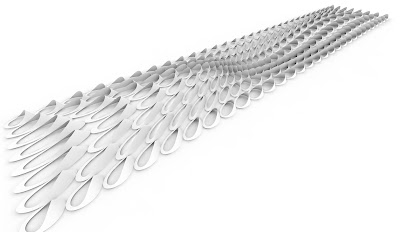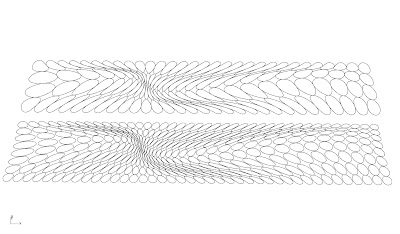My first attemp in my last parametric work has been a deformed distribuition of one component on a surface. The task was to control a deformation of one parametric component on a plane surface trough a cage rhino command. In GH this command doesn't exist yet, but i tried to mimic this useful type of deformation trough twice flow along surface component in GH. The effect seems like work...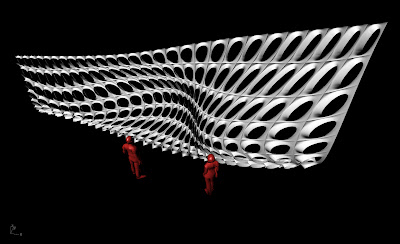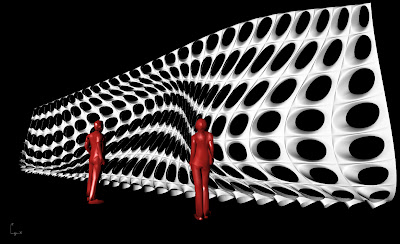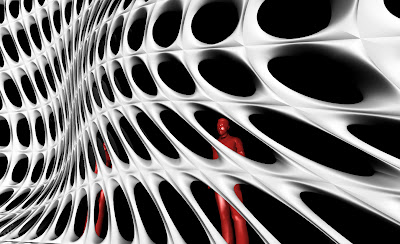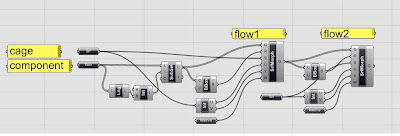## 08 November 2009

### Phyllotaxis System 05///////////////////////////////////////////////////////////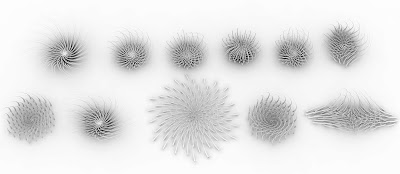An exercise about Phyllotaxis variation using VB script code in Grasshopper, working on variables with a few changes of the values "i" in the math formula of spiral: x= cos(i), y=sin(i), z=i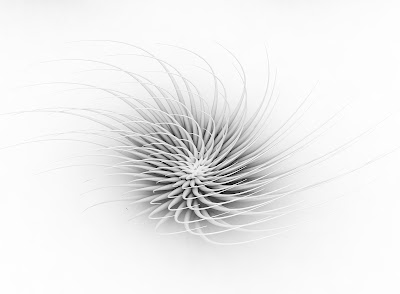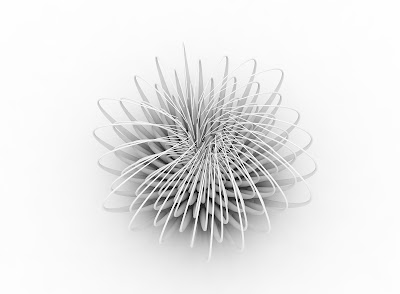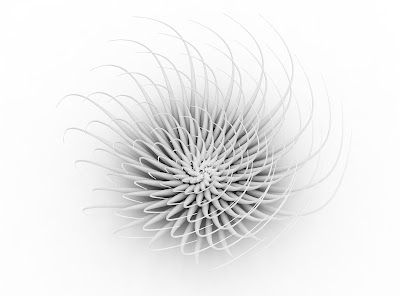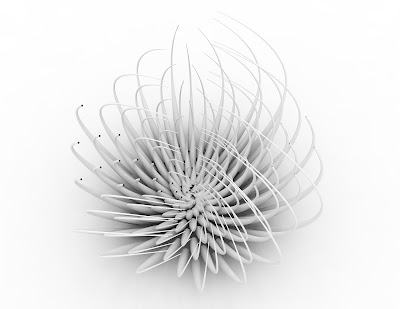"In mathematics, a spiral is a curve which emanates from a central point, getting progressively farther away as it revolves around the point.(via wikipedia)"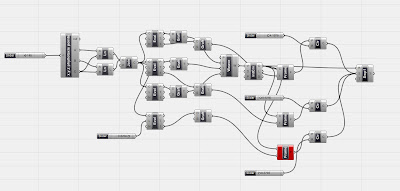Here the VB code that plots the spiral points:

Sub RunScript(ByVal n As Integer)

Dim r_list As New List (Of on3dpoint)

Dim p_list As New List (Of on3dpoint)
Dim q_list As New List (Of on3dpoint)

For i As int32=1 To n

Dim r As New on3dpoint(cos(i) * i, sin(i) * i, i / 1000)

Dim p As New on3dpoint(cos(i + 0.5) * i, sin(i + 0.5) * i, i + 1 / 1000)
Dim q As New on3dpoint(cos(i) * (i + 1), sin(i) * (i + 1), i / 1000 + (i + 4))

Next

A = r_list
B = p_list

C = q_list

End SubAnd the Rhinoscript code, but it's a wip...
///////
Dim i,arrpoint1,arrPoint2,strSphere

For i=0 To 500

arrPoint1 = Array(cos(i)*i,sin(i)*i,i/1000)
arrPoint2 = Array((cos(i+1)*i),(sin(i+1)*i)+1,(i+1/1000))